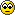# EIGRP Metrics

Hi,

I'm having a little trouble getting my head around the K values, in particular K5, and would be grateful if anyone could help clear it up.

From my understanding a K value determines how much effect a particular value has on a part of the metric calculation.
• K1 effects the weight of Bandwidth - which is manually configured on the interface;
• K2 effects the weight of Load - which I assume is calculated by the router in real time?;
• K3 effects the weight of Delay - again manually configured on the interface;
• K4 effects the weight of Reliability - is this a real time value?;
What does K5 do? I see some people saying it is MTU, but then the OCG says that MTU is not part of the metric calculation. There seems to be a bit of differing opinion on this.

Thanks.
Current objective: CCNA Security
My blog: mybraindump.co.uk

• k5 is MTU but MTU was never a part of metric calculation.You must have read some books which says MTU is used in FD calculation but its wrong.
Moreover by default in cisco IOS FD is calculated with k1 and k3 whereas k2,k4 = 0.
This is because if we use Load or reliability as a metric calculation this may cause in change of metric in every few seconds,so we avoid using them but you can still change their values if you want to practise it in your labs** K values r just integers between 1-255, just used in the formula during matric calculation. If k1 = 1 it will multiply Bandwidth by 1 , if k1 = 2 ( 2 X Bandwidth) so on.
• Member Posts: 2,008 ■■■■■■■■■□
K5 is not MTU. If K5 is 0, the last part of the equation that considers reliability is not used. It's basically an on/off switch for the optional part of the equation.
IPSec VPN Design 44%
Mastering VMWare vSphere 5​ 42.8%
• From what I read so far, K5 is a reference for MTU but was left out of the metric calculation, probably because it wouldn't effect the metric calculation productively.
• @ Zartanasaurus :- K5 is a constant used for MTU but since MTU is not a parts of Metric calculation it is not used. You can assign any value to k5 i.e from 0-255, supposse you set is to be 1 there wont be any difference in metric calculation. If you say k5 = reliability then there should be some difference in calculations that is if i make k5 = 1 or any possitive number to 255.So k5 is only for MTU not reliability.

Edit:- i agree with Zartanasaurus. k4 & k5 are integers used in metric calculations and their values tells if reliability will be included in the formula or not.• From what I understand of the maths, zartanasaraus' reasoning would seem to suit.

If the equation is:

metric = [K1 * bandwidth + (K2 * bandwidth) / (256 - load) + K3 * delay] * [K5 / (reliability + K4)]

Then K5 would be related to be reliability. 0 / anything = 0, so if I set K5 to 0 then regardless of K4 and reliability, I get 0 for that part of the equation.

Actually I've just confused myself more - in my OP I said that K2 was a multiplier for load - looking at the equation, both K1 and K2 effect bandwidth?

So load and reliability are both values generated by the router real-time by monitoring the link - and that's why it's recommended not to use them as they will change?
Current objective: CCNA Security
My blog: mybraindump.co.uk
• Ha. Nevermind. If K2 is 0 then that fraction is turned off, which gets rid of load.

Dumb moment.Current objective: CCNA Security
My blog: mybraindump.co.uk
• Member Posts: 2,008 ■■■■■■■■■□
I think we can all agree that Cisco devised this formula to confuse everyone.